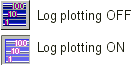Semi-log buttonToggle the log plot button to chart against a logarithmic price scale. There are two methods for displaying the price scale along the y-axis: arithmetic and logarithmic. An ARITHMETIC price scale (or linear price scale) displays a 10 dollar price range as the same vertical distance regardless of the price value. Each unit of measure is the same no matter what price you're plotting. For instance, if a stock advances from 10 to 80, the move from 10 to 20 will appear to be the same vertical distance as the move from 70 to 80. Even though this move is the same in absolute terms, it is not the same in percentage terms. A LOGARITHMIC price scale measures price movements in percentage terms. An advance from 10 to 20 would represent an increase of 100%. An advance from 20 to 40 would also be 100%, as would an advance from 40 to 80. All three of these advances would appear as the same vertical distance on a logarithmic scale. Strictly speaking, it is more accurate to refer to this mode as semi-log plotting because the horizontal scale (whether it be volume-based as in Insider TA's box charts, or time-based for more traditional charts) is still plotted linearly.